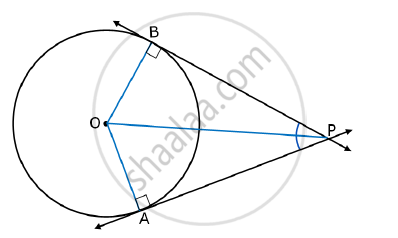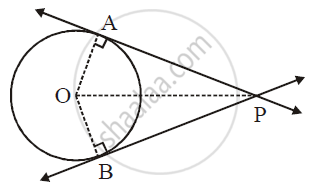# Prove that “The Lengths of the Two Tangent Segments to a Circle Drawn From An External Point Are Equal.” - Geometry

Prove that “The lengths of the two tangent segments to a circle drawn from an external point are equal.”

#### Solution 1

Given: A circle with centre O and an external point P are given.
AP and BP are the two tangents drawn from an external point P.To prove: AP = BP
Construction: Draw seg OA, seg OB and seg OP.
Proof: In ΔOBP and ΔOAP,
OA = OB … (Radii of the same circle)
OP = OP … (Side common to both the triangles)
∠OAP = ∠OBP = 90° … (tangent is perpendicular to the radius at the point of contact)
ΔOBP ≅ ΔOAP … (By R.H.S)
∴ AP = BP … (corresponding sides of congruent triangles)
Thus, the lengths of two tangent segments to a circle drawn from an external point are equal.

#### Solution 2Given: O is the centre of the circle and P is a point in the exterior of the circle. A and B are the points of contact of the two tangents from P to the circle.

To Prove: PA = PB

Construction: Draw seg OA, seg OB and seg OP.

Proof: Line AP ⊥ radius OA and line BP ⊥ radius OB   ... (Tangent perpendicular to radius)

∴ anglePAO = anglePBO = 90^@

In right-angled triangles triangle OAP and triangleOBP

hypotenuse OP ≅ hypotenuse OP    ...(Common side)

seg OA ≅ seg OB        ...(Radii of the same circle)

:.triangleOAP ≅ triangleOBP    ...(Hypotenuse-side of theorem)

∴ seg PA ≅ seg PB     ...(c.s.c.t.)

∴ PA = PB

Concept: Number of Tangents from a Point on a Circle
Is there an error in this question or solution?
2015-2016 (March) Set A

Share